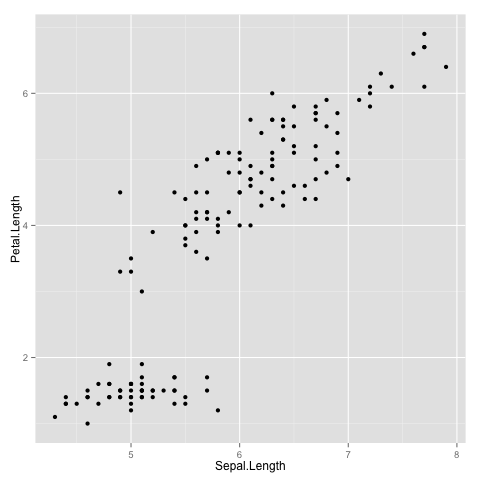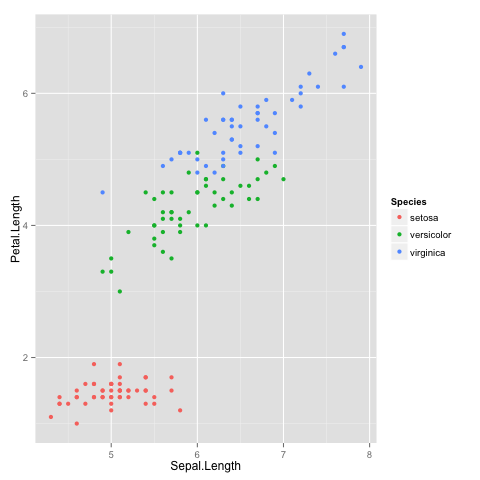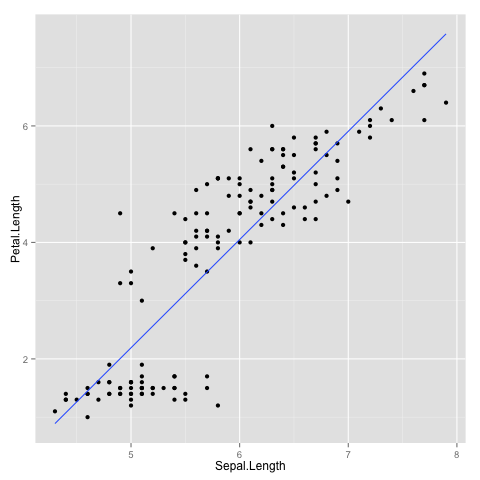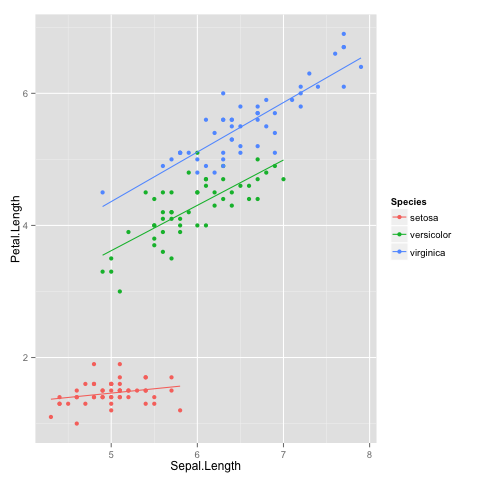In :
%load_ext rmagic


## Introduction to ggplot2¶

This is a short demo on how to convert an R Markdown Notebook into an IPython Notebook using knitr and notedown.

In :
def f(x):
return x + 2
f(2)

Out:
4

This is an introduction to ggplot2. You can view the source as an R Markdown document, if you are using an IDE like RStudio, or as an IPython notebook, thanks to notedown.

We need to first make sure that we have ggplot2 and its dependencies installed, using the install.packages function.

Now that we have it installed, we can get started by loading it into our workspace

In :
%%R
library(ggplot2)


We are now fully set to try and create some amazing plots.

#### Data¶

We will use the ubiqutous iris dataset.

In :
%%R

  Sepal.Length Sepal.Width Petal.Length Petal.Width Species
1          5.1         3.5          1.4         0.2  setosa
2          4.9         3.0          1.4         0.2  setosa
3          4.7         3.2          1.3         0.2  setosa
4          4.6         3.1          1.5         0.2  setosa
5          5.0         3.6          1.4         0.2  setosa
6          5.4         3.9          1.7         0.4  setosa


#### Simple Plot¶

Let us create a simple scatterplot of Sepal.Length with Petal.Length.

In :
%%R
ggplot(iris, aes(x = Sepal.Length, y = Petal.Length)) + geom_point()The basic idea in ggplot2 is to map different plot aesthetics to variables in the dataset. In this plot, we map the x-axis to the variable Sepal.Length and the y-axis to the variable Petal.Length.

Now suppose, we want to color the points based on the Species. ggplot2 makes it really easy, since all you need to do is map the aesthetic color to the variable Species.

In :
%%R
ggplot(iris, aes(x = Sepal.Length, y = Petal.Length)) + geom_point(aes(color = Species))Note that I could have included the color mapping right inside the ggplot line, in which case this mapping would have been applicable globally through all layers. If that doesn't make any sense to you right now, don't worry, as we will get there by the end of this tutorial.

We are interested in the relationship between Petal.Length and Sepal.Length. So, let us fit a regression line through the scatterplot. Now, before you start thinking you need to run a lm command and gather the predictions using predict, I will ask you to stop right there and read the next line of code.

In :
%%R
ggplot(iris, aes(x = Sepal.Length, y = Petal.Length)) + geom_point() + geom_smooth(method = "lm",
se = F)If you are like me when the first time I ran this, you might be thinking this is voodoo! I thought so too, but apparently it is not. It is the beauty of ggplot2 and the underlying notion of grammar of graphics.

You can extend this idea further and have a regression line plotted for each Species.

In :
%%R
ggplot(iris, aes(x = Sepal.Length, y = Petal.Length, color = Species)) + geom_point() +
geom_smooth(method = "lm", se = F)